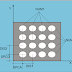## SEARCH PROGRAM

Sinumerik CYCLE 801 can be utilized to machine a "matrix gaps" drilling example. The kind of drill gap is controlled by the drilling cycle that has just been called modally.

CYCLE801 ( SPCA , SPCO ,sTA , DIS1 , DIS2 , NUM1 , NUM2 )

Where SPCA =  Reference point of a grid of hole in the abscissa (x-axis)
SPCO =  Reference point of a grid of hole in the ordinate (y-axis)
STA = Angle to abscissa (x-axis)
DIS1= Distance between columns
DIS2 = Distance between row
NUM1 = Number of column
NUM2 = Number of rows

Example :- Without angle STA=0

Dot matrix consists of 16 holes in 4 rows and 4 columns. And we call  CYCLE 81 for drilling with CYCLE 801 DOT MATRIX . A depth of drill is 30 mm.

HARSH.MPF
N10   G90 G71 G94 F50 ;
N20   T03 D01 M06 ;
N30   S1000  M03  M08;
N40   G17 G00 X15 Y15 Z50 ;
N50   MCALL CYCLE 81 (10 , 0 , 5 , -30 , 0 )
N60   CYCLE 801 ( 20 , 15, 0 , 20 , 20 , 4 , 4 )
N70   MCALL ;
N80   G00 X10 Y10 Z50 ;
N90   M05 , M09 , M30 ;

DESCRIPTION

HARSH.MPF- Name of the main program
N10- Absolute coordinate system, a metric input command, feed rate per minute 50;
N20- tool change command select tool no. 3
N30- Spindle on clockwise, speed is 1000 rpm, Coolant on.
N40- Machining in XY-plane, rapid traverse where X 15, Y15 & Z50 ( tool take position )
N50- Modal call CYCLE 81 ( RTP= 10 , RFP= 0 , SDIS = 5 , DP= -30 , DPR =0 )
N60- CALL DOT MATRIX (SPCA= 20 , SPCO = 15 , STA= 0 , DIS1= 20 , DIS2= 20 , NUM1= 4 , NUM2= 4 )
N70- Modal call
N80- Rapid traverse where tool goes X10 ,.Y10 & Z50 ;
N90- Spindle stop , Coolant off, main program end

SIEMENS SINUMERIK CYCLE 801 Dot matrixReviewed by www.hdknowledge.om on March 20, 2019 Rating: 5